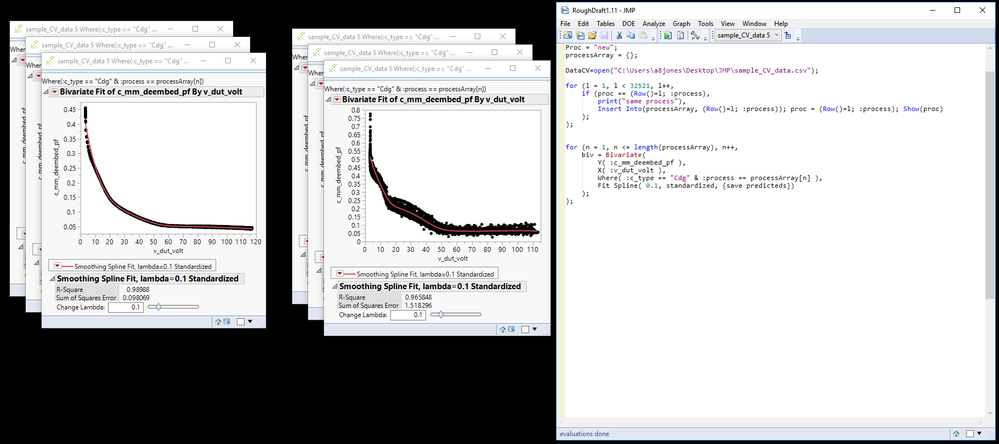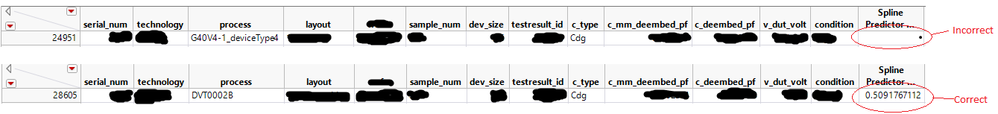Choose Language Hide Translation Bar
Highlighted

## JSL - How to iterate over Bivariate and save all splines to 1 column

Hello.  I have created an array of strings, containing the various values found in one of my columns. I would like to generate a spline for every value that is in my array of strings, and save all predicted points to a new column.  I am able to iterate over Bivariate(), changing a where-clause with each iteration to a new value in my array of strings, however, only the last predicted points are saved to a new column.  How do I get all predicted points to be saved into a new column?  Thanks.

``````Proc = "new";
processArray = {};

DataCV=open("C:\Desktop\JMP\sample_data.csv");

for (l = 1, l < 32521, l++,		// Generate array of strings
if (proc == (Row()=l; :process),
print("same process"),
Insert Into(processArray, (Row()=l; :process)); proc = (Row()=l; :process); Show(proc)
);
);

for (n = 1, n <= length(processArray), n++,	// Generate and save multiple predicteds
biv = Bivariate(
Y( :c_mm_deembed_pf ),
X( :v_dut_volt ),
Where( :c_type == "Cdg" & :process == processArray[n] ),// :process == processArray[n] appears to be working, as 6 splines are generated
Fit Spline( 0.1, standardized, {save predicteds})	// {save predicteds} is not saving all 6 splines, only last spline
);
);``````1 ACCEPTED SOLUTION

Accepted Solutions
Highlighted

## Re: JSL - How to iterate over Bivariate and save all splines to 1 column

Here is an annotated reworked version of your script.  Try it out.  I believe it will work with your data.  I was not able to do a real good job of testing, but any errors should be minor

``````Names Default To Here( 1 );
Proc = "new";
processArray = {};

DataCV = Open( "C:\Desktop\JMP\sample_data.csv" );

// In the commented area is a reworked version of your code that I believe
// simplifies the syntax of what you were doing.  Please review it and see
/*for (l = 1, l <= N Rows(DataCV) , l++,		// Generate array of strings
if (proc == :process[l],
print("same process")
,
Insert Into(processArray, :process[l]);
proc = :process[l];
Show(proc)
);
);*/

// The summarize() function is a much faster way to get the values found for
// a spcific column or combination of columns
Summarize( DataCV, processArray = By( :process ) );

For( n = 1, n <= Length( processArray ), n++, 	// Generate and save multiple predicteds
// I found that using a JSL variable in the where clause was having issues, because the formula
// created for the Predicted values contains a reference to PredictedArray, which JSL Variable can change,
// which makes the formula invalid.  So what needs to be done, is to subsitute the actual value for
// predictedArray[n] into the Where Clause
Eval(
Substitute(
Expr(
biv = Bivariate(
Y( :c_mm_deembed_pf ),
X( :v_dut_volt ),
Where( :c_type == "Cdg" & :process == __process__ ), // :process == processArray[n] appears to be working, as 6 splines are generated
Fit Spline( 0.1, standardized, {save predicteds} )	// {save predicteds} is not saving all 6 splines, only last spline
)
),
Expr( __process__ ), processArray[n]
)
)
);``````
Jim
4 REPLIES 4
Highlighted

## Re: JSL - How to iterate over Bivariate and save all splines to 1 column

Here is an annotated reworked version of your script.  Try it out.  I believe it will work with your data.  I was not able to do a real good job of testing, but any errors should be minor

``````Names Default To Here( 1 );
Proc = "new";
processArray = {};

DataCV = Open( "C:\Desktop\JMP\sample_data.csv" );

// In the commented area is a reworked version of your code that I believe
// simplifies the syntax of what you were doing.  Please review it and see
/*for (l = 1, l <= N Rows(DataCV) , l++,		// Generate array of strings
if (proc == :process[l],
print("same process")
,
Insert Into(processArray, :process[l]);
proc = :process[l];
Show(proc)
);
);*/

// The summarize() function is a much faster way to get the values found for
// a spcific column or combination of columns
Summarize( DataCV, processArray = By( :process ) );

For( n = 1, n <= Length( processArray ), n++, 	// Generate and save multiple predicteds
// I found that using a JSL variable in the where clause was having issues, because the formula
// created for the Predicted values contains a reference to PredictedArray, which JSL Variable can change,
// which makes the formula invalid.  So what needs to be done, is to subsitute the actual value for
// predictedArray[n] into the Where Clause
Eval(
Substitute(
Expr(
biv = Bivariate(
Y( :c_mm_deembed_pf ),
X( :v_dut_volt ),
Where( :c_type == "Cdg" & :process == __process__ ), // :process == processArray[n] appears to be working, as 6 splines are generated
Fit Spline( 0.1, standardized, {save predicteds} )	// {save predicteds} is not saving all 6 splines, only last spline
)
),
Expr( __process__ ), processArray[n]
)
)
);``````
Jim
Highlighted

## Re: JSL - How to iterate over Bivariate and save all splines to 1 column

And, I just thought again about the Predicted formula for the Predicted column, and realized that the process you are using is going to write on top of each other as they are processed.  I suggest that you rename the Prediced column after each execution of bivariate, forcing each iteration to end up creating a new column.

Jim
Highlighted

## Re: JSL - How to iterate over Bivariate and save all splines to 1 column

Oops. I did not see this later post and answered an earler question using Eval Expr instead of Substitute.

I didn't mean to duplicate a response.

Highlighted

## Re: JSL - How to iterate over Bivariate and save all splines to 1 column

Try the By() role to get all predicteds in the same column. Iteration over a list is then not necessary and the generated prediction formula will automatically apply conditional statements to separate the different values of the by-column. The main difference is that all plots will share the same window.

``````DataCV = Open("C:\Desktop\JMP\sample_data.csv");

DataCV << biv = Bivariate(
Y(:c_mm_deembed_pf),
X(:v_dut_volt),
By(:process),
Where(:c_type == "Cdg"),
Fit Spline(0.1, Standardized, {Save Predicteds})
);``````
Article Labels

There are no labels assigned to this post.